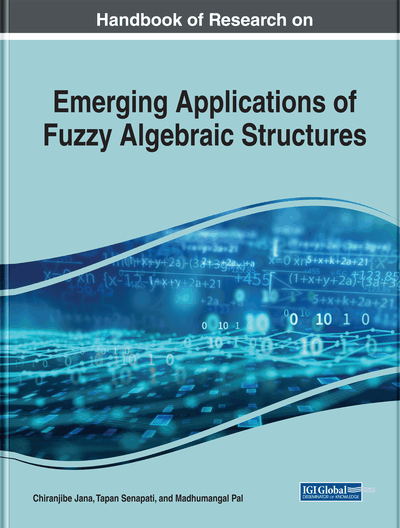Intuitionistic Fuzzy Soft Ideals

Shuker Khalil (University of Basrah, Iraq)
DOI: 10.4018/978-1-7998-0190-0.ch006
Available
\$37.50
No Current Special Offers

Abstract

The basic notions of soft sets theory are introduced by Molodtsov to deal with uncertainties when solving problems in practice as in engineering, social science, environment, and economics. This notion is convenient and easy to apply as it is free from the difficulties that appear when using other mathematical tools as theory of theory of fuzzy sets, rough sets, and theory of vague sets. The soft set theory has recently gaining significance for finding rational and logical solutions to various real-life problems, which involve uncertainty, impreciseness, and vagueness. The concepts of intuitionistic fuzzy soft left almost semigroups and the intuitionistic fuzzy soft ideal are introduced in this chapter, and some of their basic properties are studied.
Chapter Preview
Top

1. Introduction

The basic notions of soft sets theory are introduced by (Molodtsov, 1999) to deal with uncertainties when solving problems in practice as in engineering, social science, environment, and economics. This notion is convenient and easy to apply as it is free from the difficulties that appear when using other mathematical tools as theory of theory of fuzzy sets, rough sets and theory of vague sets etc. The soft set theory has recently gaining significance for finding rational and logical solutions to various real life problems, which involve uncertainty, impreciseness, and vagueness. The concepts of intuitionistic fuzzy soft left almost semigroups and the intuitionistic fuzzy soft ideal are introduced in this chapter, and some of their basic properties are studied. The fuzzy set (FS) as proposed by (Zadeh, 1965) is a framework to encounter uncertainty, vagueness, and partial truth; an FS represents the degree of membership for each member of the universe of discourse to a subset of the said universe. By adding the degree of non-membership to a fuzzy set (Atanassov,1986) proposed the intuitionistic fuzzy set (IFS), which appears more accurate for uncertainty quantification and provides opportunities to precisely model a problem based on existing knowledge and observations. The concept of an LA-semigroup was first introduced by (Kazim and Naseerudin, 1972). A non-empty set S with binary operation * is said to be a left almost semigroup (LA-semigroup), if the identity (x * y) * z = (z * y) * x for all x,y,zS holds. Subsequently, Mushtaq and others have further investigated this structure and added several useful concepts to the theory of LA-semigroups. The soft set concept was proposed by (Molodtsov, 1999) as a completely new approach for modeling and uncertainty. A soft set is a parameterized family of subsets of the universal set. (Maji et al., 2001) presented the definition of fuzzy soft set. Next, many applications of soft and fuzzy sets are shown (Aygunoglu and Aygun, 2009) introduced the concept of a fuzzy soft group and discussed some of its properties. (Maji et al. 2001, 2004) introduced the intuitionistic fuzzy soft set theory, which is based on a combination of the intuitionistic fuzzy set and soft set models. (Shuker, 2014) generated the(induced fuzzy soft set by (H,A) and(induced intuitionistic fuzzy soft set by HA), where (H,A) is a soft set over the universe of the given set with a fixed set of parameters, and HA is a fuzzy soft set. The concept of a soft set was also studied by other mathematicians. (Yaqoob et al., 2012) applied soft set theory to Γ-hyperideals in left almost Γ-semihypergroups, as also discussed see (Yaqoob et al., 2012, 2013). Moreover, (Feng et al., 2008) defined soft semirings and several related notions to establish a connection between soft sets and semirings. (Ali and Shabir, 2009) defined soft ideals and generalized fuzzy ideals in semigroups. A special structure satisfying this intuitionistic fuzzy soft algebra is given, and the relation between this structure and the theory of fuzzy soft sets is studied.In the present work, the concepts of intuitionistic fuzzy soft LA-semigroups and the intuitionistic fuzzy soft ideal are introduced, and some of their basic properties are studied.

Complete Chapter List

Search this Book:
Reset